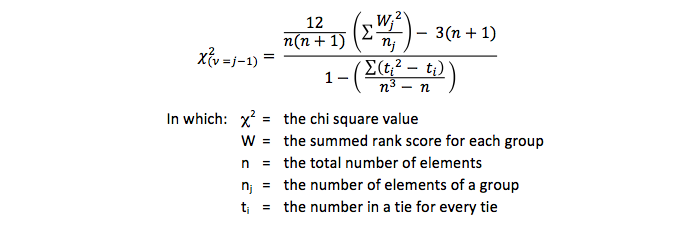# Research-Coaches.com

## Kruskal-Wallis test

### The Kruskal-Wallis test is used for comparing the mean of three groups on one variable measured on an ordinal scale.

The Kruskal-Wallis test is an extension of the Mann-Whitney test (or is the Mann-Whitney test a special case of the Kruskal-Wallis test?). Whatever. The text below has a lot of similarities.

A variable measured at ordinal, interval or ratio level can be ranked from high to low. The best gets number 1 and the second best gets number 2 and so on. These ranking scores can be divided into three or more groups. Now the question rises if one group has a higher mean than the other group. To test this statistically a Kruskal-Wallis test is applied.

## Examples of research questions for the Kruskal-Wallis test

This test is used for research questions like

• Do children in the ages of 12 to 18 run at different speeds?
• Do people in the Netherlands, Finland and Austria judge the weather differentl
• Do households with zero, one, two, three, four or more children use the same amount of electricity?

To test these questions two variables are needed.

The first one is the grouping variable with three or more values. This is the independent variable. In the first research question this is age (seven values for each age-cohort). In the second research question this is country (three values for The Netherlands, Finland and Austria). In the third research question this is type of household (four values: 1, 2, 3, 4 or more children). This grouping variable is always a variable measured at a nominal scale. If there are only two values the Mann-Whitney test should be applied.

The second one is the dependent variable. This variable is measured at an ordinal, interval or ratio scale. Variables measured at an interval or ratio scale can be transformed into ordinal variables. Therefore the Mann-Whitney test can be applied on these kinds of data too. In the first research question this can be operationalized as the time in seconds to run 100 meters. Afterwards the scores are transformed into fastest, second, third and so on. For the second research question the answers were collected on a five point rating scale, with 1 = very bad to 5 very good (a Likert scale). This is already a variable at ordinal level and it doesn’t have to be transformed. The amount of used electricity is the dependent variable in the third research question. This has to be transformed to a variable on ordinal level as well.

## The calculations of the Kruskal-Wallis test

After ranking the scores they are divided over the groups. Next these ranking scores are added for each group. If the sums of the added scores are equal for all groups, there is no difference between the groups. (This is only true if the sizes of both groups are equal.) To test if the summed scores differ between groups a W-value is calculated as the square of the sum score divided by the number of elements in that group. This Summed W-score is tested with this formula:However if ties exist (a tie is an equal rank score) this formula should be used:Statisticians recommend to have at least 30 respondents / subjects / objects in each group. With larger group sizes - say above 30 each - an ANOVA is recommended.

## Related topics to Kruskal-Wallis test